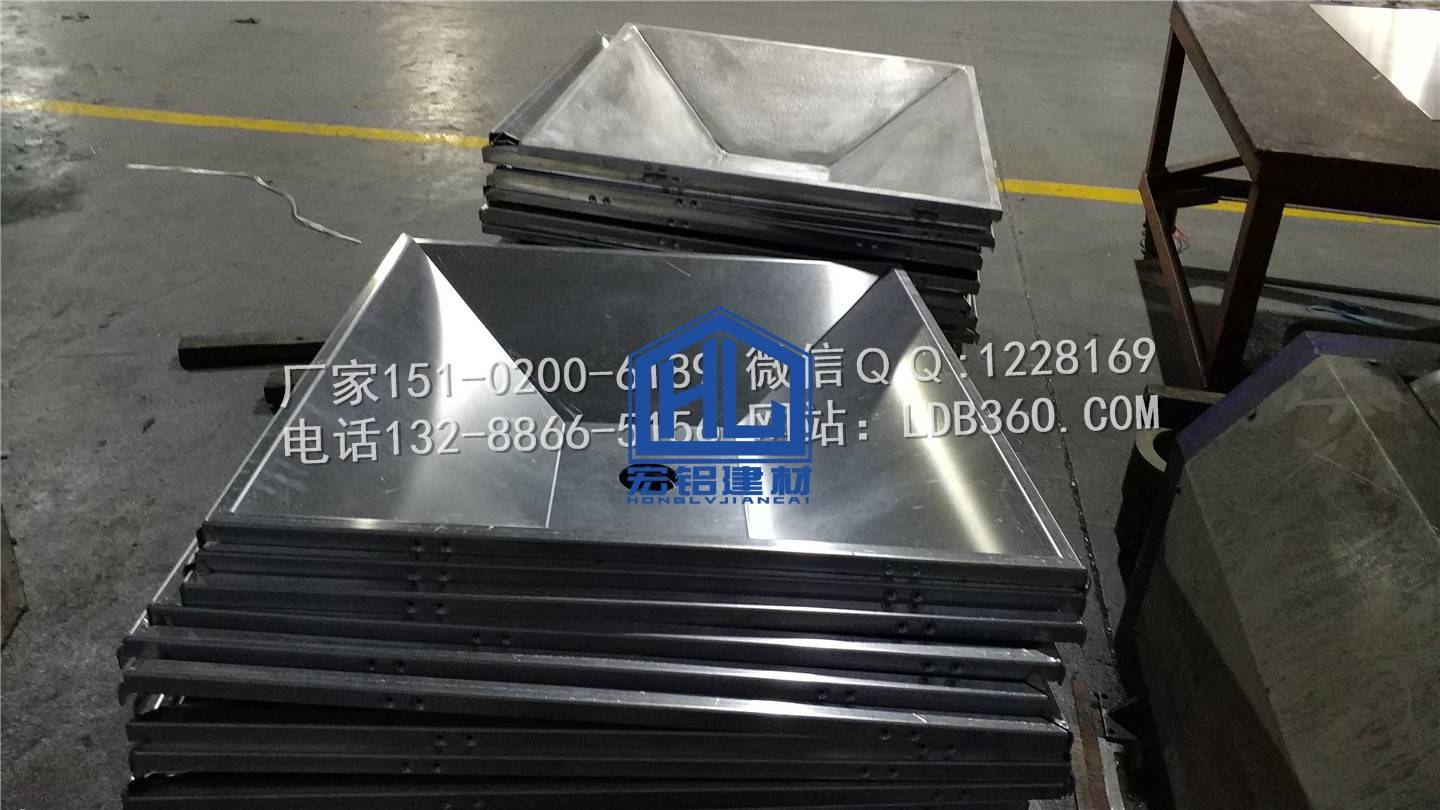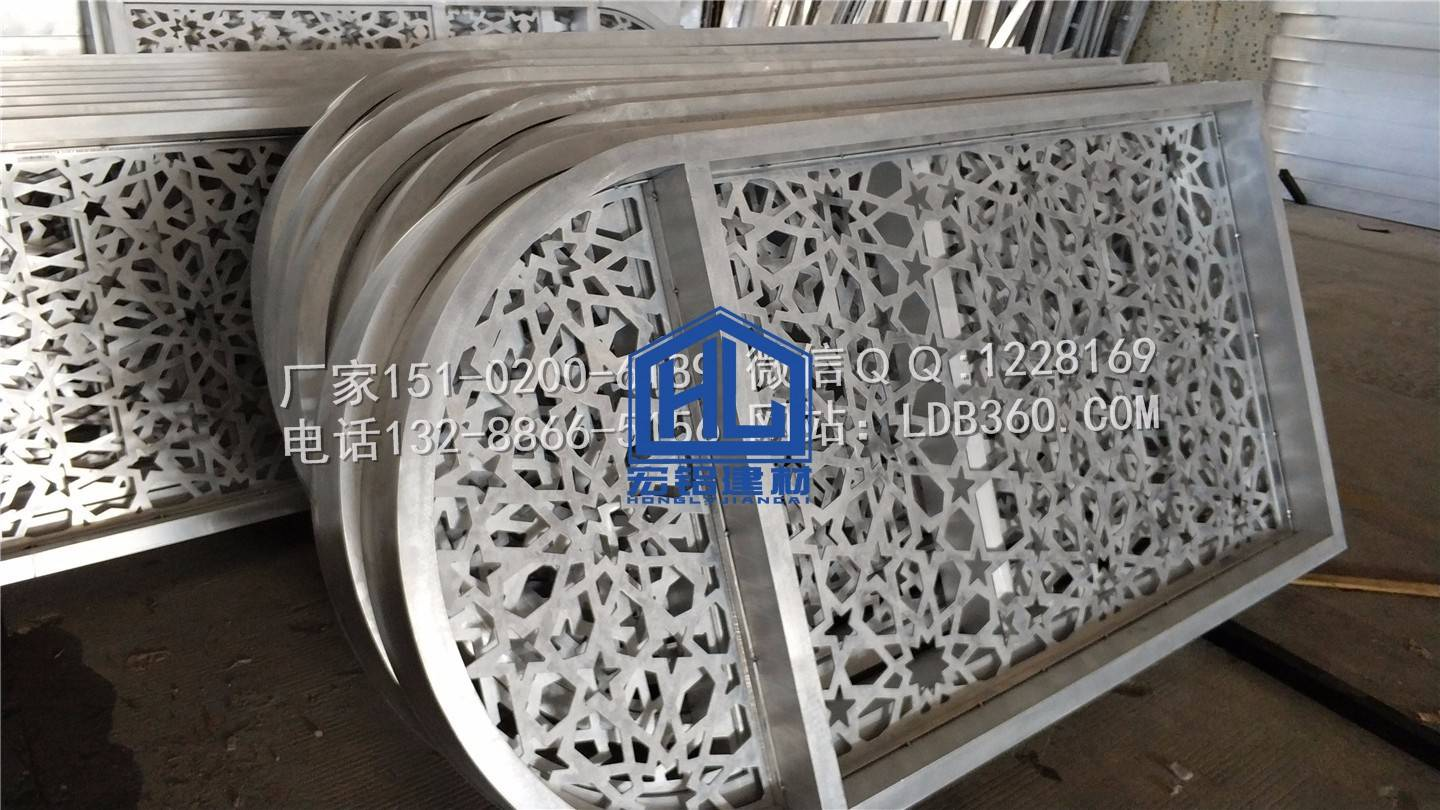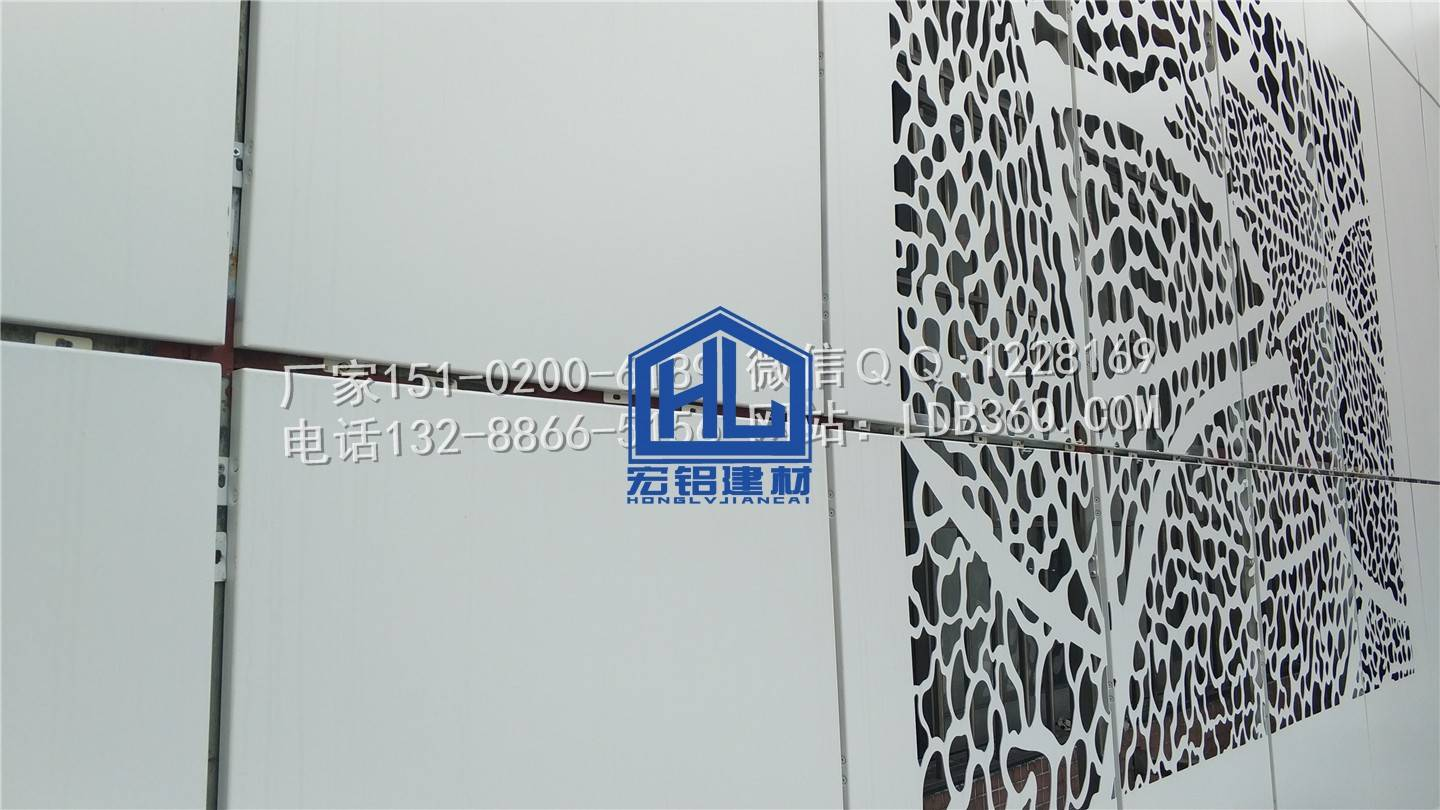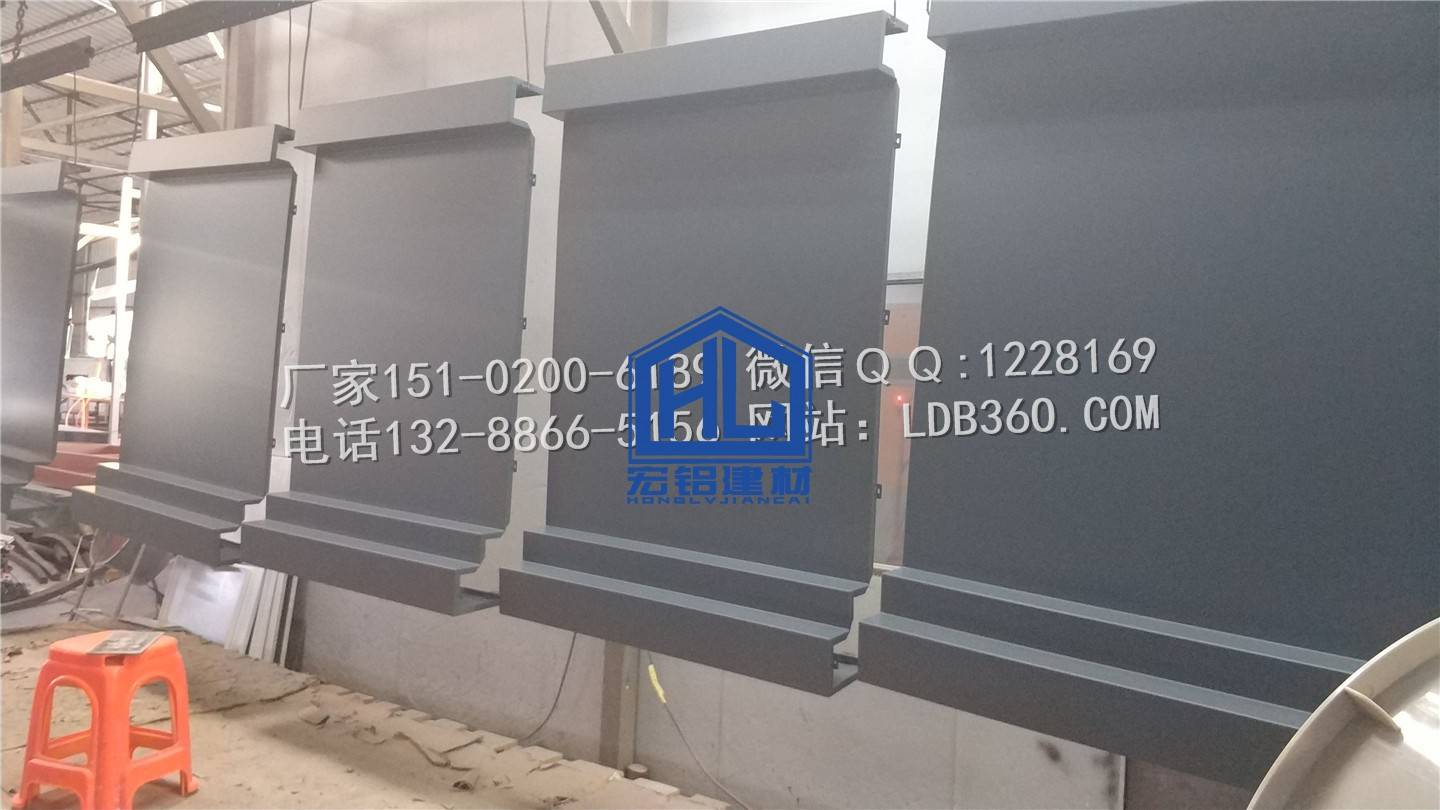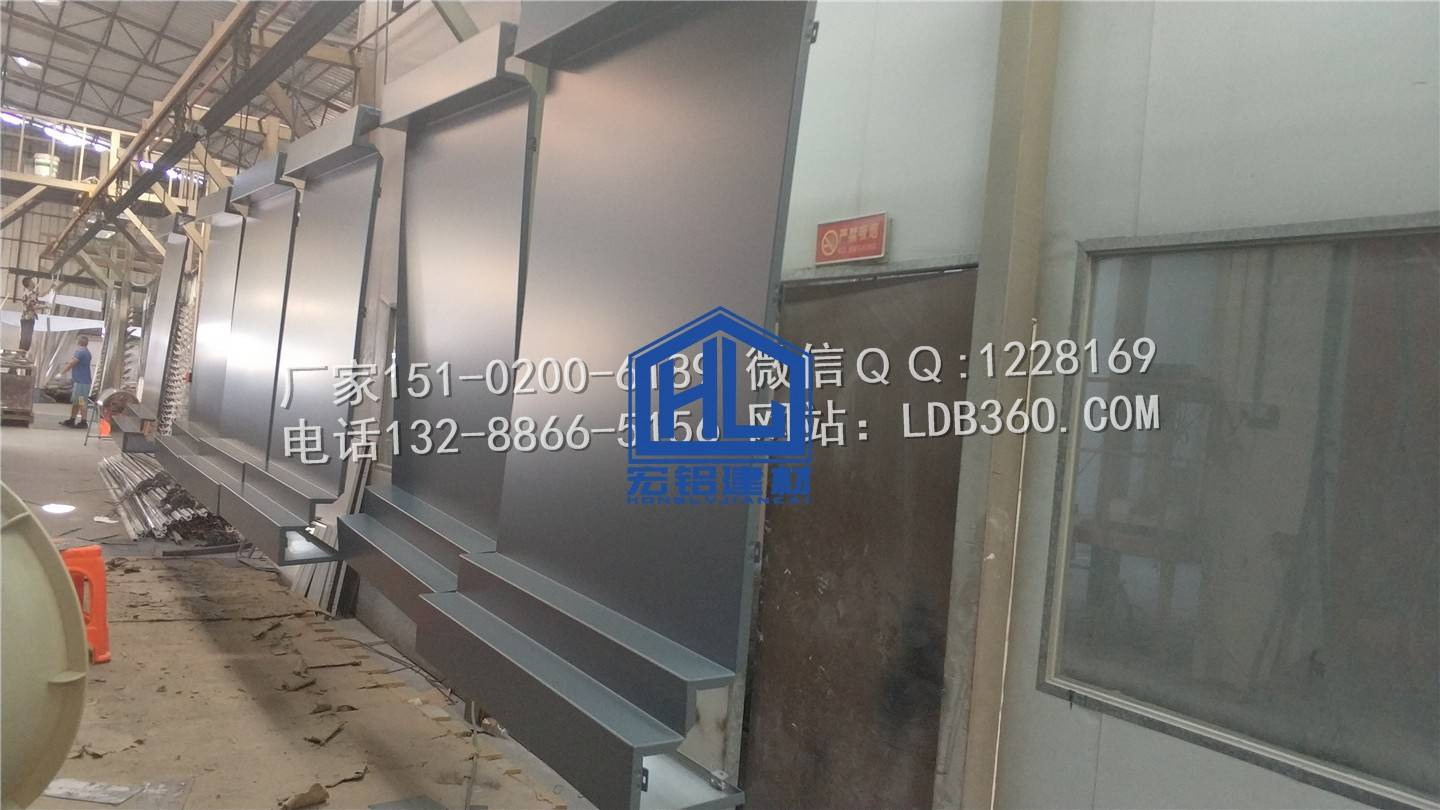# Bending coefficient of aluminum veneer, and calculation of opening size of aluminum plate!

2020-11-29 15:27:06 宏铝建材 9326
 Bending coefficient table of aluminum veneer Thickness of aluminum plate (Mm) Bending angle (°) Bending coefficient (Mm) Zero point eightMm Ninety One point five OneMm Ninety One point five 45、135 Zero point five One point twoMm Ninety Two 45、135 Zero point five One point fiveMm Ninety Two point five 45、135 Zero point five 60、120 One point five TwoMm Ninety Three 45、135 One 60、120 Two point five Ninety degree slotting One point five Two point fiveMm Ninety Four 45、135 One point five 60、120 Three Ninety degree slotting Two Threemm 90 Five 45、135 Three 60、120 Four point five 90 degree slotting Two point five There are many calculation methods for bending coefficient, such as K-factor method, Y-factor method and plate thickness deduction method, and the common method is bending deduction number method. That is: unfold size = a straight edge length + B straight edge length - bending coefficient, these formulas are commonly used in our production cutting!For example: the length and width are 1000mm, the four sides are 90 degrees, and the folding edge is 20mmFor ordinary aluminum veneer, many people think that the opening size is 1040 * 1040mmHowever, the actual cutting is 1034 * 1034mm. Because of the 90 degree bending of 2.0, the bending coefficient without slotting is 3.0 mm, and the length and width of simply supported plates on four sides are bent by two knives, and the cutting size should be reduced by 6 mm